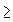## Project 7: Disjoint Triangles

Suppose we are given 3n points in the plane (n1), no three of which are collinear (i.e. lie on a straight line).

Problems

1. Is it possible to create from these points n triangles which have no points in common (i.e. are disjoint)?

2. Given 6 points in the plane no three of which are collinear, for what configuration(s) of the points is the number of ways to form two disjoint triangles a maximum? a minimum?

3. If there is a configuration of 6 points for which one can select k pairs of three points and form two disjoint triangles and another 6 point configuration for which one can select m pairs of three points and form two disjoint triangles are there 6 point configurations for which this can be done for each number which lies between k and m?

3. How does the situation change if, instead of insisting that the triangles formed have no points in common, we require that the convex hulls of the triangles have no points in common?

Extensions

1. Extend Problem 2 to 9, 12, etc., points.

2. Generalize problem 3.

3. Generalize the problems above to (s)(n) points.

Joseph Malkevitch
Department of Mathematics and Computing
York College (CUNY)
Jamaica, New York 11451-0001
email: malkevitch@york.cuny.edu
(Comments and results related to the project above are welcome.)

Acknowledgements
Some of this work was prepared with partial support from the National Science Foundation (Grant Number: DUE 9555401) to the Long Island Consortium for Interconnected Learning (administered by SUNY at Stony Brook, Alan Tucker, Project Director).